### 2.3.1.2 Forward value iteration

The ideas from Section 2.3.1 may be recycled to yield a symmetrically equivalent method that computes optimal cost-to-come functions from the initial stage. Whereas backward value iterations were able to find optimal plans from all initial states simultaneously, forward value iterations can be used to find optimal plans to all states in. In the backward case,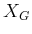must be fixed, and in the forward case,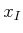must be fixed.

The issue of maintaining feasible solutions appears again. In the forward direction, the role of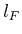is not important. It may be applied in the last iteration, or it can be dropped altogether for problems that do not have a predetermined. However, one must force all plans considered by forward value iteration to originate from. We again have the choice of either making notation that imposes constraints on the action spaces or simply adding a term that forces infeasible plans to have infinite cost. Once again, the latter will be chosen here.

Let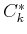denote the optimal cost-to-come from stageto stage, optimized over all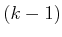-step plans. To preclude plans that do not start at, the definition of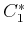is given by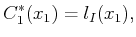(2.13)

in which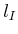is a new function that yields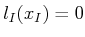, and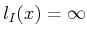for all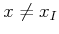. Thus, any plans that try to start from a state other thanwill immediately receive infinite cost.

For an intermediate stage,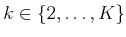, the following represents the optimal cost-to-come: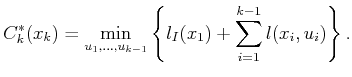(2.14)

Note that the sum refers to a sequence of states,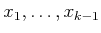, which is the result of applying the action sequence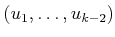. The last state,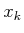, is not included because its cost term,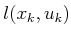, requires the application of an action,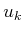, which has not been chosen. If it is possible to write the cost additively, as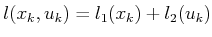, then the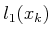part could be included in the cost-to-come definition, if desired. This detail will not be considered further.

As in (2.5), it is assumed in (2.14) that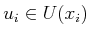for every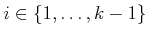. The resulting, obtained after applying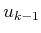, must be the samethat is named in the argument on the left side of (2.14). It might appear odd that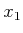appears inside of the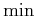above; however, this is not a problem. The statecan be completely determined once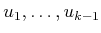andare given.

The final forward value iteration is the arrival at the final stage,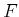. The cost-to-come in this case is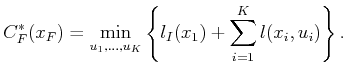(2.15)

This equation looks the same as (2.5) after substituting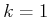; however,is used here instead of. This has the effect of filtering the plans that are considered to include only those that start at. The forward value iterations find optimal plans to any reachable final state from. This behavior is complementary to that of backward value iteration. In that case,was fixed, and optimal plans from any initial state were found. For forward value iteration, this is reversed.

To express the dynamic-programming recurrence, one further issue remains. Suppose that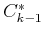is known by induction, and we want to compute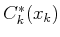for a particular. This means that we must start at some state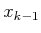and arrive in stateby applying some action. Once again, the backward state transition equation from Section 2.2.3 is useful. Using the stage indices, it is written here as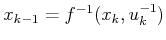.

The recurrence is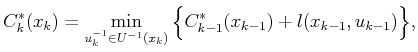(2.16)

in whichand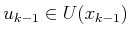is the input to which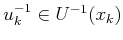corresponds. Using (2.16), the final cost-to-come is iteratively computed in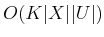time, as in the case of computing the first-stage cost-to-go in the backward value-iteration method.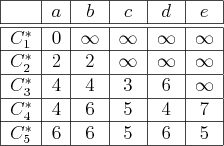Example 2..4 (Forward Value Iteration)   Example 2.3 is revisited for the case of forward value iterations with a fixed plan length of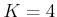. The cost-to-come functions shown in Figure 2.12 are obtained by direct application of (2.16). It will be helpful to refer to Figures 2.10 and 2.11 once again. The first row corresponds to the immediate application of. In the second row, finite values are obtained for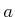and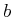, which are reachable in one stage from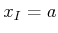. The iterations continue until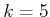, at which point that optimal cost-to-come is determined for every state.Steven M LaValle 2020-08-14